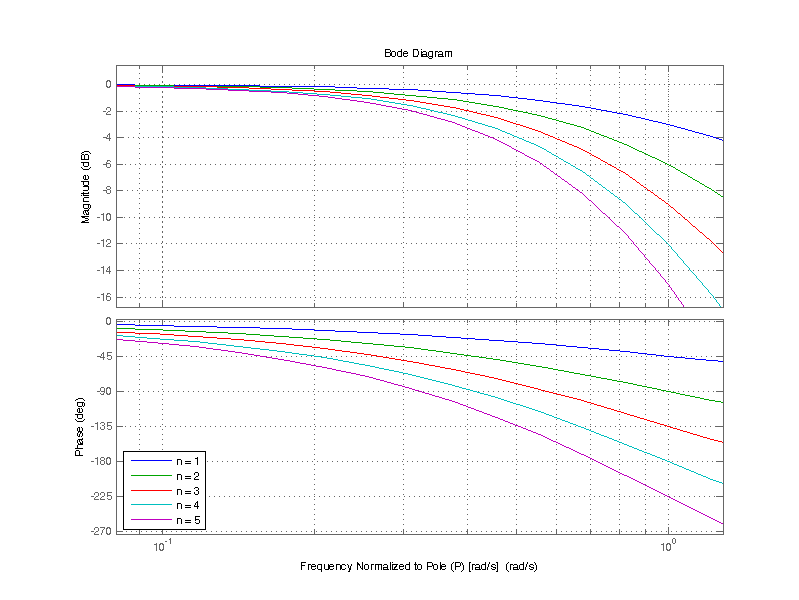One approach to improving stopband rejection is to cascade several first-order filters, there by achieving the combined rejection of n filters for better stopband rejection. While the implantation of such an arrangement is straight forward, there are some consequences to this approach which will be discussed below.

For a low-pass filter of the form

$H(s) = \dfrac{1}{1 + s/p}$

From control theory the cascaded transfer function is just the multiplication of each filter transform. When each filter has an identical transfer function the overall transfer function is the following

$C(s) = \dfrac{1}{(1 + s/p)^n}$

The poles of C(s) are easy to compute, as there are n identical roots of the denominator at s = -p. C(s) has n poles at

$p_1 = p_2 = … = p_n = -p$

Applying the binomial theorem to the denominator yields

$C(s) = \dfrac{1}{1 + {n\choose 1}\left( \dfrac{s}{p} \right) + {n\choose 2}\left( \dfrac{s}{p} \right)^2 +…+ {n\choose n}\left( \dfrac{s}{p} \right)^n }$

From the expansion of C(s) the first major draw back can be seen that the first s term is multiplied by n, which results in moving the -3 dB point from fc to towards DC.

Frequency Response of H(jw)
Normalized Frequency (w/p) n=1 Magnitude (dB) n=2 Magnitude (dB)  n=3 Magnitude (dB)  n=4 Magnitude (dB)  n=5 Magnitude (dB)
0.01 -0.0 -0.0 -0.0 -0.0 -0.0
0.1 -0.0 -0.1 -0.1 -0.2 -0.2
1 -3.0 -6.0 -9.0 -12.0 -15.1
10 -20.0 -40.1 -60.1 -80.2 -100.2
100 -40.0 -80.0 -120.0 -160.0 -200

A bode plot of the combined transfer function for 1 to 5 cascaded single pole filters is shown below.Note that the -3 dB attenuation at $$\omega_c$$ is cumulative for n cascaded poles, i.e. for n=5 the attenuation at $$\omega_c$$ is -15 dB.Consequently, the effective bandwidth as defined by the -3 dB point decreases for an increasing number of cascaded single pole filters. The effective bandwidth when 1 to 5 single pole filters are cascaded is shown in the table below.

Effective bandwidth of cascading first order low-pass filters
Number of Filters Bandwidth
1 fc
2 0.64*fc
3 0.51*fc
4 0.43*fc
5 0.38*fc

In the case of cascading 5 single pole filters, one could instead use 5 filters with a corner frequency 2.63*fc, where fc is the desired -3 dB corner frequency. However the attenuation in the first frequency decade of the stopband is not simple n times the single pole case. The table below shows the filter attenuation, for the frequency one decade past the -3 dB point.